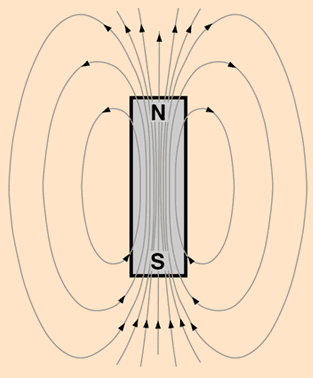# Magnetic field "lines" confused with magnetic field "vectors"

• I
I might be a slow learner, but am still trying to understand the difference between field lines and vectors.

I've got that magnetic field lines are symbolic and that the directional arrows applied (from north to south) are a convention.

But see the attached image. The field lines form a closed loop and while within the north pole point in the same direction as the magnetic field vector. But while in the south pole of the magnet, the direction of the field line, is in the opposite direction of the field vector.

Assuming my descriptions are correct, what does this tell us about the convention of magnetic field lines and field vectors?

Thank you.

#### Attachments

•Magnetic-Field-Lines-Vector-Forces.jpg
65.6 KB · Views: 222
Last edited by a moderator:

BvU
Homework Helper
But while in the south pole of the magnet, the direction of the field line, is in the opposite direction of the field vector
No. Both go from norh to south

Not exactly sure what you mean BvU.
So would that mean my diagram is incorrect and if so do the magnetic field vectors from the north and south pole both point in the same direction or do the magnetic field lines change direction somehow when shown inside one half of the magnet??
TIA.

nasu
Gold Member
The magnetic field vector at the south pole is drawn the wrong way. It should enter the magnet.

Thanks nasu.
Does that mean the direction for the red magnetic field vector, which we call the south pole should be flipped 180 degrees? That would mean it's pointing in the same direction as the blue north pole, you sure this is correct?

sophiecentaur
Gold Member
2020 Award
Basically a line only has direction. A vector has direction and magnitude. The magnitude corresponds to the density of the lines in a region. The directions are the same - why would they not be as they correspond to the path that an 'isolated N pole' would take?

nasu
Gold Member
Thanks nasu.
Does that mean the direction for the red magnetic field vector, which we call the south pole should be flipped 180 degrees? That would mean it's pointing in the same direction as the blue north pole, you sure this is correct?
Yes, the arrow at the south pole is in the wrong direction. The arrows on the lines simply show the direction of a magnetic field vector tangent to the line for any point on the line.

Thank you for your input, I can see where my misunderstanding is derived. Thanks for the image robphy, I got my own compass and magnets out and indeed the direction of the field lines go in the one direction. Iv'e drawn the field lines correctly, but it seems not the magnetic field vectors. But that now introduces a new misunderstanding hopefully you can help me with.

Sliding a paper clip along a bar magnet and it's obvious there's very little magnetic force in the middle, while it's strongest at the ends. My understanding was that the magnetic field vectors for the domains in a ferromagnetic material would point in opposite directions in the north and south pole. This would explain why adding the vectors from the domains close to the middle of the magnet would summate to zero, hence why there's so little force detected there. While at the ends, the poles are sufficiently separated so as to not influence each other.

Obviously this is not the case, hopefully someone can provide a proper explanation? TIA.

robphy
Homework Helper
Gold Member
http://hyperphysics.phy-astr.gsu.edu/hbase/magnetic/elemag.html

Note that there is big difference between the inside of a bar magnet and the outside of a bar magnet when near the center of the magnet. Look at the "density of field lines" in this diagram taken from aboveHigher density of field lines means that the field is stronger there.

For domains, look at
http://hyperphysics.phy-astr.gsu.edu/hbase/Solids/ferro.html#c4